# Olympiad Test: Alphabet Test - 2

## 20 Questions MCQ Test Mathematical Olympiad Class 8 | Olympiad Test: Alphabet Test - 2

Description
Attempt Olympiad Test: Alphabet Test - 2 | 20 questions in 40 minutes | Mock test for Class 8 preparation | Free important questions MCQ to study Mathematical Olympiad Class 8 for Class 8 Exam | Download free PDF with solutions
QUESTION: 1

### Direction: Arrange the given words in alphabetical order and choose the one that comes first.

Solution:

Qualify, Quality, Quarrel, Quarter

QUESTION: 2

### Direction: Arrange the given words in alphabetical order and choose the one that comes first.

Solution:

Science, Secure, Security, Service

QUESTION: 3

### Direction: Arrange the given words in alphabetical order and choose the one that comes first.

Solution:

Social, Society, Sock, Soil

QUESTION: 4

Direction: Arrange the given words in alphabetical order and choose the one that comes first.

Solution:

Discipline, Disney, Disorder, Distroy.

QUESTION: 5

Direction: Arrange the given words in alphabetical order and choose the one that comes first.

Solution:

Centre, Consent, Constant, Cotton.

QUESTION: 6

Direction: Arrange the given words in the alphabetical order and choose the word that comes last.

Solution:

Accurate, Agriculture, Assignment, Attention

QUESTION: 7

Direction: Arrange the given words in the alphabetical order and choose the word that comes last.

Solution:

Registrar, Registration, Restriction, Resume

QUESTION: 8

Direction: Arrange the given words in the alphabetical order and choose the word that comes last.

Solution:

Hill, Holiday, Honest, Hunter

QUESTION: 9

Direction: Arrange the given words in the alphabetical order and choose the word that comes last.

Solution:

Test, Train, Treat, Triple.

QUESTION: 10

Direction: Arrange the given words in the alphabetical order and choose the word that comes last.

Solution:

Eager, Earn, Else, Empty

QUESTION: 11

Direction: Each of the following question is based on the following alphabet series.
A B C D E F G H I J K L M N O P Q R S T U V W X Y Z.
Q. Which letter is fifth to the right of eighth letter from the right end of the alphabet?

Solution: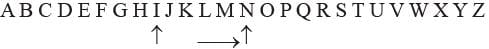QUESTION: 12

Direction: Each of the following question is based on the following alphabet series.
A B C D E F G H I J K L M N O P Q R S T U V W X Y Z.
Q. If the order of English alphabet is reversed then which letter would be exactly in the middle?

Solution:

Z Y X W V U T S R Q P O N M L K J I H G F E D C B A

The middle letter is N and M

QUESTION: 13

Direction: Each of the following question is based on the following alphabet series.
A B C D E F G H I J K L M N O P Q R S T U V W X Y Z.
Q. If only first half of the given alphabet is reversed, how many letters will be there between J and S?

Solution: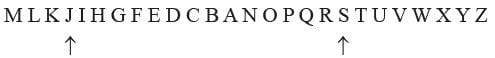QUESTION: 14

Direction: Each of the following question is based on the following alphabet series.
A B C D E F G H I J K L M N O P Q R S T U V W X Y Z.
Q. Which letter in the alphabet is as far from G as T is from M?

Solution:

A B C D E F G H I J K L M N O P Q R S T U V W X Y Z

QUESTION: 15

Direction: Each of the following question is based on the following alphabet series.
A B C D E F G H I J K L M N O P Q R S T U V W X Y Z.
Q. If the given alphabet is written in the reverse order then which letter would be sixth letter to the right of P?

Solution: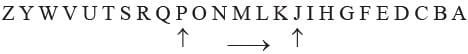QUESTION: 16

Direction: Each of the following question is based on the following alphabet series.
A B C D E F G H I J K L M N O P Q R S T U V W X Y Z.
Q. If the second half of the given alphabet is reversed then which letter will be seventh letter to the right of K?

Solution: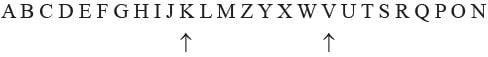QUESTION: 17

Direction: Each of the following question is based on the following alphabet series.
A B C D E F G H I J K L M N O P Q R S T U V W X Y Z.
Q. If first ten letters of the given alphabet is reversed then which letter will be 4th letter to the right of L?

Solution: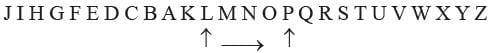QUESTION: 18

Direction: Each of the following question is based on the following alphabet series.
A B C D E F G H I J K L M N O P Q R S T U V W X Y Z.
Q. Which letter will be exactly midway between P and Z?

Solution: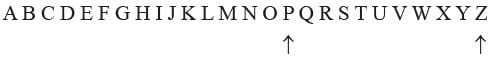QUESTION: 19

Direction: Each of the following question is based on the following alphabet series.
A B C D E F G H I J K L M N O P Q R S T U V W X Y Z.
Q. Which letter will be exactly midway between N and V if the alphabet is written in reverse order?

Solution: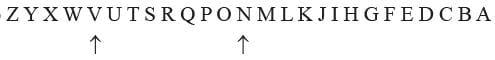QUESTION: 20

Direction: Each of the following question is based on the following alphabet series.
A B C D E F G H I J K L M N O P Q R S T U V W X Y Z.
Q. Which letter will be the fifth to the left of 17th letter from the right end of the alphabet?

Solution: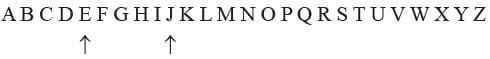Use Code STAYHOME200 and get INR 200 additional OFF Use Coupon Code